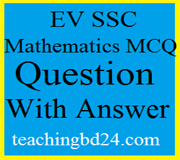# EV SSC MCQ Question Ans. Practical Geometry

EV SSC MCQ Question Ans. Practical Geometry. In  the  previous classes, geometrical   figures  were  drawn  in  proving  different propositions and in the exercises. There was no need for precision in drawing these figures.  But sometimes  precision  is  necessary  for  geometrical  constructions.  oFr example, when an architect makes a design of a house or an engineer draws different parts  of  a  machine,  high  precision  of  drawing  is  required.  In such  geometrical constructions, one makes use of ruler and compasses only. We have already learned how to construct triangles and quadrilaterals with the help of ruler and compasses. In this  chapter,   we  will  discuss  the  construction   of  some  special  triangles  and quadrilaterals.

## EV SSC MCQ Question Ans. Practical Geometry###Every triangle has three sides and three angles. But, to specify the shape and size of a triangle, all sides and angles need not be specified. oFr example, as the sum of the three angles of a triangle, is two right angles, one can easily find the measurement of the third angle when the measurement of the two angles of the triangle given. Again, from  the  theorems  on  congruence  of triangles,  it  is  found  that  the  following combination  of  three  sides  and angles  are  enough  to  be  congruent.  That  is,  a combination of these three parts of a triangle is enough to construct a unique triangle.

teachingbd24.com is such a website where you would get all kinds of necessary information regarding educational notes, suggestions and questions’ patterns of school, college and madrasahs. Particularly you will get here special notes of physics that will be immensely useful to both students and teachers. The builder of the website is Mr. Md. Shah Jamal Who has been serving for 30 years as an Asst. Professor of BAF Shaheen College. He expects that this website will meet up all the needs of Bengali version learners /students. He has requested concerned both students and teachers to spread this website home and abroad.